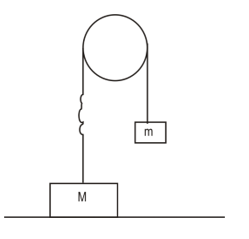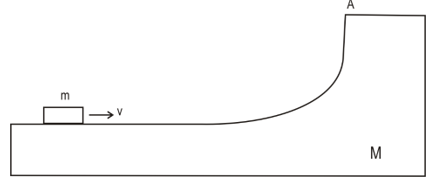# Linear momentum Questions

In this page we have Momentum Questions for JEE Main ,Advanced and NEET . Hope you like them and do not forget to like , social share and comment at the end of the page.

## Multiple choice Questions

Question 1
A 5kg rifle fires a 6g bullet with Speed of 500m/s. Find the ratio of the distance the rifle move backward while the bullet is in the barrel to the distance the bullet moves forward?
(a) 1/600
(b) 1/666
(c) 2/327
(d) none of the above

Question 2
A neutron of mass m is collides elastically with a nucleus of mass M, which is initially at rest. Neutron kinetic energy is k0. Find the maximum kinetic energy it can lose
(a) $\frac {2mMK_0}{(M+m)^2}$
(b) $\frac {4mMK_0}{(M+m)^2}$
(c) $\frac {mMK_0}{(M+m)^2}$
(d) none of the above

(A)A person Jack stands at left end of a railway car sitting on  a railway track. There is no frictional force between the track and the railway car.
Person + Railway track Mass =M
Length of the railway car         =L
Jack throws a ball of mass m with velocity u towards the right end of the railway car where it collides elastically with the wall and travel back to the other end and strike the wall inelastically  and coming to rest.
i is the unit vector across right end.
Question 3
Match the column
Column A
(P) Velocity of the railway car after the first collision
(Q) Velocity of the railway car after the second collision
(R) Velocity of the railway car before first collision
(S) Velocity of the Center of the mass of system( Railway car + jack + ball)
Column B
(A) 0
(B)$\frac {-m}{M} u \mathbf{i}$
(C)$\frac {m}{M} u \mathbf{i}$
(D)$\frac {-m}{M+m} u \mathbf{i}$

Question 4
Which of the following statement is true
(a) The ball will take $\frac {L}{(1+ \frac {m}{M})u}$ time to strike the right end
(b) The ball will $\frac {2L}{(1+ \frac {m}{M})u}$ time to strike the left end
(c) There will be no net displacement of the railway car at the end of time $\frac {2L}{(1+ \frac {m}{M})u}$
(d) There will no change in center of the mass position of the system (Railway car + jack + ball)

(B) After falling from rest through a distance h, a body of mass m begin to raise a body of Mass M (M >m) that is connected to it by means of a light inextensible string passing over a fixed smooth pulleyQuestion 5
Find the velocity of the mass after the jerk
(a)$\frac{M}{m}\sqrt{2gh}$
(b) $\frac{M}{m+M}\sqrt{2gh}$
(c) $\frac{m}{M}\sqrt{2gh}$
(d)$\frac{m}{m+M}\sqrt{2gh}$

Question 6
Find the time taken for the body of mass M to return to its original position
(a)$\frac{2m}{M-m}\sqrt{\frac{2h}{g}}$
(b)$\frac{2M}{M-m}\sqrt{\frac{2h}{g}}$
(c)$\frac{2m}{M}\sqrt{\frac{2h}{g}}$
(d) None of these

Question 7
Find the fraction of the KE lost after the first Jerk
(a) $\frac{M}{m+M}$
(b) $\frac{m}{m+M}$
(c) $\frac{m}{M}$
(d) None of these

(C)A missile of mass M moving with velocity v= 200m/sec explodes in mid air breaking into two parts of mass M/4, 3M/4. If the smaller piece flies off at and angle of 60° with respect to the original direction with speed 400m/s.

Question 8
Find the velocity of the second piece
(a) 100 m/s
(b) 250 m/s
(c) 300m/s
(d) 231 m/s

Question 9
Q is defined as
$Q=KE_f - KE_i$
What is the value of Q in this case?
(a) $\frac {23361M}{8}$
(b)$\frac {13361M}{8}$
(c)$\frac {53361M}{8}$
(d) none of the above

(D) A particle of mass m moves such a way that momentum vector varies as
$\boldsymbol{p(t)}=\mathbf{i}+t(1-at)\mathbf{j}$
Where i and j are unit vector across x and y axis

Question 10
Find the Force vector on the mass where $\mathbf{F}\bot \mathbf{p}$
(a) j
(b) –j
(c) i +j
(d) i -j

Question 11
Find the impulse on the mass over the period t and t+ $\Delta t$
(a) $\Delta t(1-a\Delta t+2a\Delta t)\mathbf{j}$
(b) $\Delta t(1+a\Delta t-2a\Delta t)\mathbf{j}$
(c) $\Delta t(a\Delta t+2a\Delta t)\mathbf{j}$
(d) $\Delta t(1-2a\Delta t)\mathbf{j}$

(E) A rocket ascends from rest in a uniform gravitational field by ejecting exhaust with constant speed u. The rate of expulsion is $\frac {dm}{dt} = \lambda m$ where m is the instantaneous mass of the rocket and λ is constant. The rocket is also retarded by air resistance with a force (mbv) where b is constant

Question 12
Find the velocity of the rocket as a function of time
(a) $v=\frac{g}{b}-\frac{e^{-bt}(u\lambda-g)}{b}$
(b) $v=1-\frac{e^{-bt}(u\lambda-g)}{b}$
(c) $v=\frac{u\lambda-g}{b}-\frac{e^{-bt}}{b}$
(d) $v=\frac{u\lambda-g}{b}-\frac{e^{-bt}(u\lambda-g)}{b}$

Question 13
Find the terminal velocity of the rocket
(a) $v=\frac{g}{b}-\frac{(u\lambda-g)}{b}$
(b) $v=\frac{g}{b}$
(c) $v=\frac{u\lambda-g}{b}$
d) $v=\frac{g-u\lambda}{b}$

## Multiple Choice Questions

Question 14
A flat car of weight W roll without resistance along a horizontal surface .Initially a car together with a 3 men of weight w each is moving to the right with v0
Two cases are considered
Case1- All the three move simultaneously with velocity u relative to floor of car toward left. And jump off at the left
Case2- All the men run with Relative Velocity u and jump in succession towards left
Which of the following is true
(a) increase in velocity of flat car will be independent of the initial v0 of the system in both cases
(b) final velocity of flat car will be more in case2
(c) increment in velocity in case1 is given by $\frac {3wu}{W + 3w}$
(d) Increment in velocity in case2 after the second man jump is $\frac {wu}{W + 2w}$

Question 15
A body of mass M with small disc of mass m placed on its rest on a smooth horizontal plane.
The disc is set in motion in horizontal motion with velocity v.which of the following is true
(a) the block M will starts moving towards due to constant force exerted by the disc m
(b) the height to which disc rise after breaking at point A from initial level is $\frac {Mv^2}{2g(M+m)}$
(c) velocity of mass M when disc break from is $\frac {mv}{M+m}$
(d) none of the above

Question 16
An object of mass M is at the origin and is at rest. Suddenly an explosion happens in the object and object is divided three equal parts.
The first part goes with the velocity (v0i+v0 j) .
The second part goes with the velocity v0 j
What is the velocity vector for the third part?
(a) (v0i+v0 j)
(b) (-v0i-2v0 j)
(c) (v0i-v0 j)
(d) None of these

Question 17
A flat car filled with sand is moving with velocity v. The flat car is leaking sand at the rate $\frac {dm}{dt}$ Find the velocity of the flat car after ½ amount has leaked
(a) v
(b) v/2
(c) v/4
(d) $v{log}_e{2}$

## Subjective Questions

Question 18
A machine gun fires six bullets per sec into a target. The mass of each bullet is 4 g.The speeds is 600m/s.Find the average force required to hold the gun in position. What is the power delivered to the bullet?

Question 19
Cannon of Mass m starts sliding freely down a smooth inclined plane at an angle α to the horizontal. After the canon has reached v0 , a shot was fired .The shell leaves the canon in horizontal direction with a momentum p1. The cannon stop as consequences.
Assume the mass of the shell is negligible as compared to the canon
(a)The change in momentum of the system
(b)Calculate the duration of the shot

Question 20
A flat car of mass m0 starts moving to the right due to constant  horizontal force F at t=0.Sand spills on the flat car from the stationary hopper .The velocity of loading is μ kg/sec.
(a) Calculate the Initial acceleration of the flat car
(b) Velocity and acceleration as a function of time
(c) Momentum of the car at any instant
(d) If the sand hopper moves with velocity v0 in the left direction, what will be the velocity of the car at any instant?

Question 21
A bullet of mass m  is fired horizontally with a velocity v on a wooden block of mass M suspended from a support  and gets embedded in it.
(a) Calculate the final velocity of the composite block
(b) Calculate the KE of the composite block
(c) Find the height reached by the composite block